Imagination is more important than knowledge... Albert Einstein

Guess is more important than calculation --- Knowhowacademy.com

Equation - System of Equations

For SAT1
Number of Total Problems: 10.
FOR PRINT ::: (Book)

 Problem Num : 1 Type: Understanding Section:Equation Theme: Adjustment# : Difficulty: 2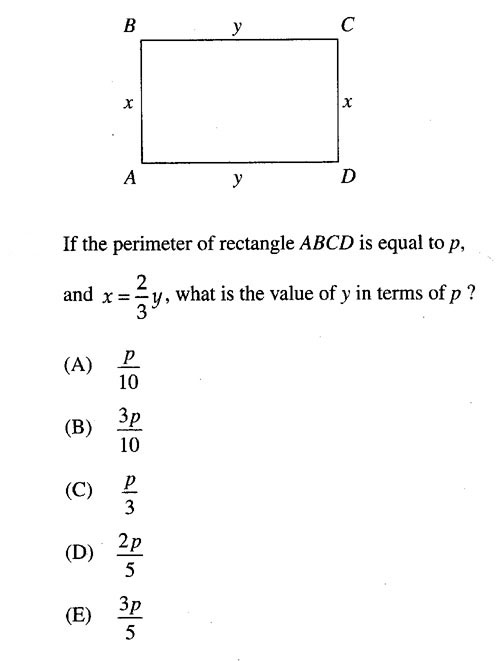Category System of Equations
Analysis

 Problem Num : 2 Type: Word problem Section:Equation Theme: Adjustment# : Difficulty: 1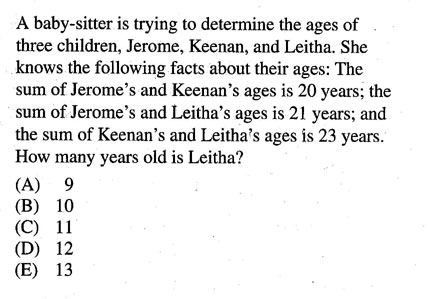Category System of Equations
Analysis

 Problem Num : 3 Type: Many Variables Section:Equation Theme: Adjustment# : Difficulty: 2Category System of Equations
Analysis ????? ?????? ??? ???? ????

 Problem Num : 4 Type: Many Variables Section:Equation Theme: Adjustment# : Difficulty: 1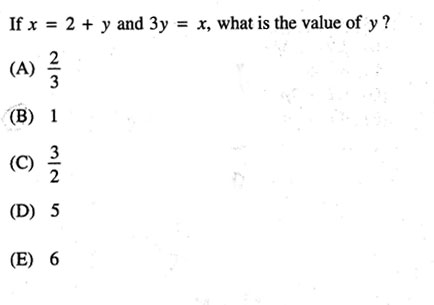Category System of Equations
Analysis

 Problem Num : 5 Type: Many Variables Section:Equation Theme: Adjustment# : Difficulty: 1Category System of Equations
Analysis

 Problem Num : 6 Type: Many Variables Section:Equation Theme: Adjustment# : Difficulty: 1Category System of Equations
Analysis

 Problem Num : 7 Type: None Section:Equation Theme: Adjustment# : Difficulty: 1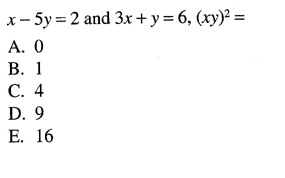Category System of Equations
Analysis

 Problem Num : 8 Type: Calculation Section:Equation Theme: Adjustment# : Difficulty: 2Category System of Equations
Analysis

 Problem Num : 9 Type: Calculation Section:Equation Theme: Adjustment# : Difficulty: 2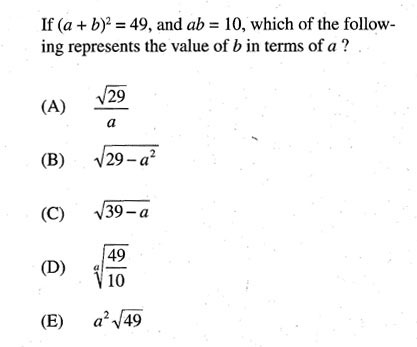Category System of Equations
Analysis

 Problem Num : 10 Type: Application Section:Equation Theme: Adjustment# : Difficulty: 2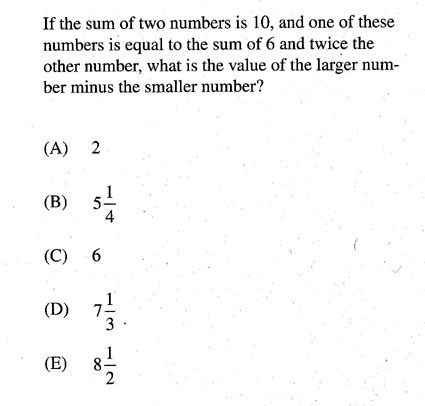Category System of Equations
Analysis You need two variables.